# 10.5 Polar form of complex numbers  (Page 4/8)

 Page 4 / 8

## Finding the n Th root of a complex number

Evaluate the cube roots of $\text{\hspace{0.17em}}z=8\left(\mathrm{cos}\left(\frac{2\pi }{3}\right)+i\mathrm{sin}\left(\frac{2\pi }{3}\right)\right).$

We have

$\begin{array}{l}{z}^{\frac{1}{3}}={8}^{\frac{1}{3}}\left[\mathrm{cos}\left(\frac{\frac{2\pi }{3}}{3}+\frac{2k\pi }{3}\right)+i\mathrm{sin}\left(\frac{\frac{2\pi }{3}}{3}+\frac{2k\pi }{3}\right)\right]\hfill \\ {z}^{\frac{1}{3}}=2\left[\mathrm{cos}\left(\frac{2\pi }{9}+\frac{2k\pi }{3}\right)+i\mathrm{sin}\left(\frac{2\pi }{9}+\frac{2k\pi }{3}\right)\right]\hfill \end{array}$

There will be three roots: $\text{\hspace{0.17em}}k=0,\text{\hspace{0.17em}}\text{\hspace{0.17em}}1,\text{\hspace{0.17em}}\text{\hspace{0.17em}}2.\text{\hspace{0.17em}}$ When $\text{\hspace{0.17em}}k=0,\text{\hspace{0.17em}}$ we have

${z}^{\frac{1}{3}}=2\left(\mathrm{cos}\left(\frac{2\pi }{9}\right)+i\mathrm{sin}\left(\frac{2\pi }{9}\right)\right)$

When $\text{\hspace{0.17em}}k=1,\text{\hspace{0.17em}}$ we have

When $\text{\hspace{0.17em}}k=2,\text{\hspace{0.17em}}$ we have

Remember to find the common denominator to simplify fractions in situations like this one. For $\text{\hspace{0.17em}}k=1,\text{\hspace{0.17em}}$ the angle simplification is

$\begin{array}{l}\frac{\frac{2\pi }{3}}{3}+\frac{2\left(1\right)\pi }{3}=\frac{2\pi }{3}\left(\frac{1}{3}\right)+\frac{2\left(1\right)\pi }{3}\left(\frac{3}{3}\right)\hfill \\ \text{\hspace{0.17em}}\text{\hspace{0.17em}}\text{\hspace{0.17em}}\text{\hspace{0.17em}}\text{\hspace{0.17em}}\text{\hspace{0.17em}}\text{\hspace{0.17em}}\text{\hspace{0.17em}}\text{\hspace{0.17em}}\text{\hspace{0.17em}}\text{\hspace{0.17em}}\text{\hspace{0.17em}}\text{\hspace{0.17em}}\text{\hspace{0.17em}}\text{\hspace{0.17em}}\text{\hspace{0.17em}}\text{\hspace{0.17em}}\text{\hspace{0.17em}}\text{\hspace{0.17em}}\text{\hspace{0.17em}}\text{\hspace{0.17em}}\text{\hspace{0.17em}}\text{\hspace{0.17em}}\text{\hspace{0.17em}}\text{\hspace{0.17em}}\text{\hspace{0.17em}}=\frac{2\pi }{9}+\frac{6\pi }{9}\hfill \\ \text{\hspace{0.17em}}\text{\hspace{0.17em}}\text{\hspace{0.17em}}\text{\hspace{0.17em}}\text{\hspace{0.17em}}\text{\hspace{0.17em}}\text{\hspace{0.17em}}\text{\hspace{0.17em}}\text{\hspace{0.17em}}\text{\hspace{0.17em}}\text{\hspace{0.17em}}\text{\hspace{0.17em}}\text{\hspace{0.17em}}\text{\hspace{0.17em}}\text{\hspace{0.17em}}\text{\hspace{0.17em}}\text{\hspace{0.17em}}\text{\hspace{0.17em}}\text{\hspace{0.17em}}\text{\hspace{0.17em}}\text{\hspace{0.17em}}\text{\hspace{0.17em}}\text{\hspace{0.17em}}\text{\hspace{0.17em}}\text{\hspace{0.17em}}\text{\hspace{0.17em}}=\frac{8\pi }{9}\hfill \end{array}$

Find the four fourth roots of $\text{\hspace{0.17em}}16\left(\mathrm{cos}\left(120°\right)+i\mathrm{sin}\left(120°\right)\right).$

${z}_{0}=2\left(\mathrm{cos}\left(30°\right)+i\mathrm{sin}\left(30°\right)\right)$

${z}_{1}=2\left(\mathrm{cos}\left(120°\right)+i\mathrm{sin}\left(120°\right)\right)$

${z}_{2}=2\left(\mathrm{cos}\left(210°\right)+i\mathrm{sin}\left(210°\right)\right)$

${z}_{3}=2\left(\mathrm{cos}\left(300°\right)+i\mathrm{sin}\left(300°\right)\right)$

Access these online resources for additional instruction and practice with polar forms of complex numbers.

## Key concepts

• Complex numbers in the form $\text{\hspace{0.17em}}a+bi\text{\hspace{0.17em}}$ are plotted in the complex plane similar to the way rectangular coordinates are plotted in the rectangular plane. Label the x- axis as the real axis and the y- axis as the imaginary axis. See [link] .
• The absolute value of a complex number is the same as its magnitude. It is the distance from the origin to the point: $\text{\hspace{0.17em}}|z|=\sqrt{{a}^{2}+{b}^{2}}.\text{\hspace{0.17em}}$ See [link] and [link] .
• To write complex numbers in polar form, we use the formulas $\text{\hspace{0.17em}}x=r\mathrm{cos}\text{\hspace{0.17em}}\theta ,y=r\mathrm{sin}\text{\hspace{0.17em}}\theta ,\text{\hspace{0.17em}}$ and $\text{\hspace{0.17em}}r=\sqrt{{x}^{2}+{y}^{2}}.\text{\hspace{0.17em}}$ Then, $\text{\hspace{0.17em}}z=r\left(\mathrm{cos}\text{\hspace{0.17em}}\theta +i\mathrm{sin}\text{\hspace{0.17em}}\theta \right).\text{\hspace{0.17em}}$ See [link] and [link] .
• To convert from polar form to rectangular form, first evaluate the trigonometric functions. Then, multiply through by $\text{\hspace{0.17em}}r.\text{\hspace{0.17em}}$ See [link] and [link] .
• To find the product of two complex numbers, multiply the two moduli and add the two angles. Evaluate the trigonometric functions, and multiply using the distributive property. See [link] .
• To find the quotient of two complex numbers in polar form, find the quotient of the two moduli and the difference of the two angles. See [link] .
• To find the power of a complex number $\text{\hspace{0.17em}}{z}^{n},\text{\hspace{0.17em}}$ raise $\text{\hspace{0.17em}}r\text{\hspace{0.17em}}$ to the power $\text{\hspace{0.17em}}n,$ and multiply $\text{\hspace{0.17em}}\theta \text{\hspace{0.17em}}$ by $\text{\hspace{0.17em}}n.\text{\hspace{0.17em}}$ See [link] .
• Finding the roots of a complex number is the same as raising a complex number to a power, but using a rational exponent. See [link] .

## Verbal

A complex number is $\text{\hspace{0.17em}}a+bi.\text{\hspace{0.17em}}$ Explain each part.

a is the real part, b is the imaginary part, and $\text{\hspace{0.17em}}i=\sqrt{-1}$

What does the absolute value of a complex number represent?

How is a complex number converted to polar form?

Polar form converts the real and imaginary part of the complex number in polar form using $\text{\hspace{0.17em}}x=r\mathrm{cos}\theta \text{\hspace{0.17em}}$ and $\text{\hspace{0.17em}}y=r\mathrm{sin}\theta .$

How do we find the product of two complex numbers?

What is De Moivre’s Theorem and what is it used for?

${z}^{n}={r}^{n}\left(\mathrm{cos}\left(n\theta \right)+i\mathrm{sin}\left(n\theta \right)\right)\text{\hspace{0.17em}}$ It is used to simplify polar form when a number has been raised to a power.

## Algebraic

For the following exercises, find the absolute value of the given complex number.

$5+\text{​}3i$

$-7+\text{​}i$

$5\sqrt{2}$

$-3-3i$

$\sqrt{2}-6i$

$\sqrt{38}$

$2i$

$2.2-3.1i$

$\sqrt{14.45}$

For the following exercises, write the complex number in polar form.

$2+2i$

$8-4i$

$4\sqrt{5}\mathrm{cis}\left(333.4°\right)$

$-\frac{1}{2}-\frac{1}{2}\text{​}i$

$\sqrt{3}+i$

$2\mathrm{cis}\left(\frac{\pi }{6}\right)$

$3i$

For the following exercises, convert the complex number from polar to rectangular form.

$z=7\mathrm{cis}\left(\frac{\pi }{6}\right)$

$\frac{7\sqrt{3}}{2}+i\frac{7}{2}$

$z=2\mathrm{cis}\left(\frac{\pi }{3}\right)$

$z=4\mathrm{cis}\left(\frac{7\pi }{6}\right)$

$-2\sqrt{3}-2i$

$z=7\mathrm{cis}\left(25°\right)$

$z=3\mathrm{cis}\left(240°\right)$

$-1.5-i\frac{3\sqrt{3}}{2}$

$z=\sqrt{2}\mathrm{cis}\left(100°\right)$

For the following exercises, find $\text{\hspace{0.17em}}{z}_{1}{z}_{2}\text{\hspace{0.17em}}$ in polar form.

$4\sqrt{3}\mathrm{cis}\left(198°\right)$

$\frac{3}{4}\mathrm{cis}\left(180°\right)$

$5\sqrt{3}\mathrm{cis}\left(\frac{17\pi }{24}\right)$

For the following exercises, find $\text{\hspace{0.17em}}\frac{{z}_{1}}{{z}_{2}}\text{\hspace{0.17em}}$ in polar form.

$7\mathrm{cis}\left(70°\right)$

$5\mathrm{cis}\left(80°\right)$

$5\mathrm{cis}\left(\frac{\pi }{3}\right)$

For the following exercises, find the powers of each complex number in polar form.

Find $\text{\hspace{0.17em}}{z}^{3}\text{\hspace{0.17em}}$ when $\text{\hspace{0.17em}}z=5\mathrm{cis}\left(45°\right).$

$125\mathrm{cis}\left(135°\right)$

Find $\text{\hspace{0.17em}}{z}^{4}\text{\hspace{0.17em}}$ when $\text{\hspace{0.17em}}z=2\mathrm{cis}\left(70°\right).$

Find $\text{\hspace{0.17em}}{z}^{2}\text{\hspace{0.17em}}$ when $\text{\hspace{0.17em}}z=3\mathrm{cis}\left(120°\right).$

$9\mathrm{cis}\left(240°\right)$

Find $\text{\hspace{0.17em}}{z}^{2}\text{\hspace{0.17em}}$ when $\text{\hspace{0.17em}}z=4\mathrm{cis}\left(\frac{\pi }{4}\right).$

Find $\text{\hspace{0.17em}}{z}^{4}\text{\hspace{0.17em}}$ when $\text{\hspace{0.17em}}z=\mathrm{cis}\left(\frac{3\pi }{16}\right).$

$\mathrm{cis}\left(\frac{3\pi }{4}\right)$

Find $\text{\hspace{0.17em}}{z}^{3}\text{\hspace{0.17em}}$ when $\text{\hspace{0.17em}}z=3\mathrm{cis}\left(\frac{5\pi }{3}\right).$

For the following exercises, evaluate each root.

Evaluate the cube root of $\text{\hspace{0.17em}}z\text{\hspace{0.17em}}$ when $\text{\hspace{0.17em}}z=27\mathrm{cis}\left(240°\right).$

$\text{\hspace{0.17em}}3\mathrm{cis}\left(80°\right),3\mathrm{cis}\left(200°\right),3\mathrm{cis}\left(320°\right)\text{\hspace{0.17em}}$

Evaluate the square root of $\text{\hspace{0.17em}}z\text{\hspace{0.17em}}$ when $\text{\hspace{0.17em}}z=16\mathrm{cis}\left(100°\right).$

Evaluate the cube root of $\text{\hspace{0.17em}}z\text{\hspace{0.17em}}$ when $\text{\hspace{0.17em}}z=32\mathrm{cis}\left(\frac{2\pi }{3}\right).$

$\text{\hspace{0.17em}}2\sqrt{4}\mathrm{cis}\left(\frac{2\pi }{9}\right),2\sqrt{4}\mathrm{cis}\left(\frac{8\pi }{9}\right),2\sqrt{4}\mathrm{cis}\left(\frac{14\pi }{9}\right)$

Evaluate the square root of $\text{\hspace{0.17em}}z\text{\hspace{0.17em}}$ when $\text{\hspace{0.17em}}z=32\text{cis}\left(\pi \right).$

Evaluate the cube root of $\text{\hspace{0.17em}}z\text{\hspace{0.17em}}$ when $\text{\hspace{0.17em}}z=8\mathrm{cis}\left(\frac{7\pi }{4}\right).$

$2\sqrt{2}\mathrm{cis}\left(\frac{7\pi }{8}\right),2\sqrt{2}\mathrm{cis}\left(\frac{15\pi }{8}\right)$

## Graphical

For the following exercises, plot the complex number in the complex plane.

$2+4i$

$-3-3i$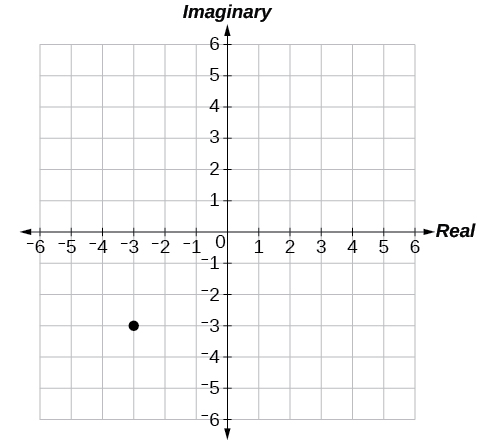$5-4i$

$-1-5i$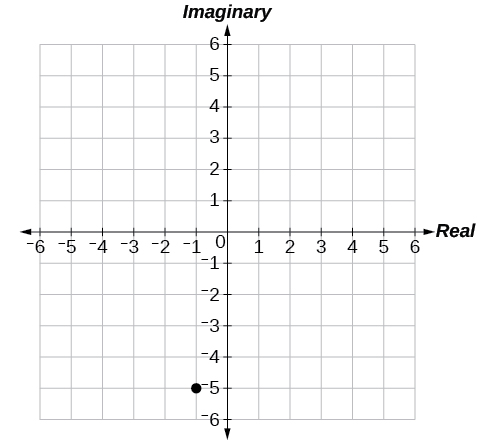$3+2i$

$2i$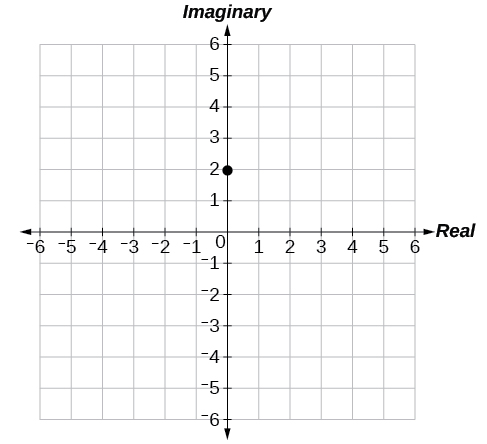$-4$

$6-2i$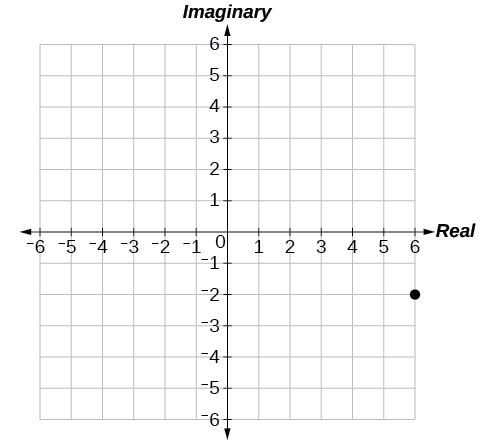$-2+i$

$1-4i$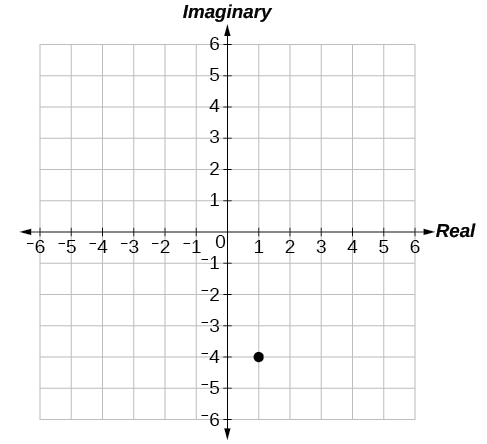## Technology

For the following exercises, find all answers rounded to the nearest hundredth.

Use the rectangular to polar feature on the graphing calculator to change $\text{\hspace{0.17em}}5+5i\text{\hspace{0.17em}}$ to polar form.

Use the rectangular to polar feature on the graphing calculator to change $\text{\hspace{0.17em}}3-2i\text{\hspace{0.17em}}$ to polar form.

$\text{\hspace{0.17em}}3.61{e}^{-0.59i}\text{\hspace{0.17em}}$

Use the rectangular to polar feature on the graphing calculator to change $-3-8i\text{\hspace{0.17em}}$ to polar form.

Use the polar to rectangular feature on the graphing calculator to change $\text{\hspace{0.17em}}4\mathrm{cis}\left(120°\right)\text{\hspace{0.17em}}$ to rectangular form.

$\text{\hspace{0.17em}}-2+3.46i\text{\hspace{0.17em}}$

Use the polar to rectangular feature on the graphing calculator to change $\text{\hspace{0.17em}}2\mathrm{cis}\left(45°\right)\text{\hspace{0.17em}}$ to rectangular form.

Use the polar to rectangular feature on the graphing calculator to change $\text{\hspace{0.17em}}5\mathrm{cis}\left(210°\right)\text{\hspace{0.17em}}$ to rectangular form.

$\text{\hspace{0.17em}}-4.33-2.50i\text{\hspace{0.17em}}$

what are you up to?
nothing up todat yet
Miranda
hi
jai
hello
jai
Miranda Drice
jai
aap konsi country se ho
jai
which language is that
Miranda
I am living in india
jai
good
Miranda
what is the formula for calculating algebraic
I think the formula for calculating algebraic is the statement of the equality of two expression stimulate by a set of addition, multiplication, soustraction, division, raising to a power and extraction of Root. U believe by having those in the equation you will be in measure to calculate it
Miranda
state and prove Cayley hamilton therom
hello
Propessor
hi
Miranda
the Cayley hamilton Theorem state if A is a square matrix and if f(x) is its characterics polynomial then f(x)=0 in another ways evey square matrix is a root of its chatacteristics polynomial.
Miranda
hi
jai
hi Miranda
jai
thanks
Propessor
welcome
jai
What is algebra
algebra is a branch of the mathematics to calculate expressions follow.
Miranda
Miranda Drice would you mind teaching me mathematics? I think you are really good at math. I'm not good at it. In fact I hate it. 😅😅😅
Jeffrey
lolll who told you I'm good at it
Miranda
something seems to wispher me to my ear that u are good at it. lol
Jeffrey
lolllll if you say so
Miranda
but seriously, Im really bad at math. And I hate it. But you see, I downloaded this app two months ago hoping to master it.
Jeffrey
which grade are you in though
Miranda
oh woww I understand
Miranda
Jeffrey
Jeffrey
Miranda
how come you finished in college and you don't like math though
Miranda
gotta practice, holmie
Steve
if you never use it you won't be able to appreciate it
Steve
I don't know why. But Im trying to like it.
Jeffrey
yes steve. you're right
Jeffrey
so you better
Miranda
what is the solution of the given equation?
which equation
Miranda
I dont know. lol
Jeffrey
Miranda
Jeffrey
answer and questions in exercise 11.2 sums
how do u calculate inequality of irrational number?
Alaba
give me an example
Chris
and I will walk you through it
Chris
cos (-z)= cos z .
cos(- z)=cos z
Mustafa
what is a algebra
(x+x)3=?
6x
Obed
what is the identity of 1-cos²5x equal to?
__john __05
Kishu
Hi
Abdel
hi
Ye
hi
Nokwanda
C'est comment
Abdel
Hi
Amanda
hello
SORIE
Hiiii
Chinni
hello
Ranjay
hi
ANSHU
hiiii
Chinni
h r u friends
Chinni
yes
Hassan
so is their any Genius in mathematics here let chat guys and get to know each other's
SORIE
I speak French
Abdel
okay no problem since we gather here and get to know each other
SORIE
hi im stupid at math and just wanna join here
Yaona
lol nahhh none of us here are stupid it's just that we have Fast, Medium, and slow learner bro but we all going to work things out together
SORIE
it's 12
what is the function of sine with respect of cosine , graphically
tangent bruh
Steve
cosx.cos2x.cos4x.cos8x
sinx sin2x is linearly dependent
what is a reciprocal
The reciprocal of a number is 1 divided by a number. eg the reciprocal of 10 is 1/10 which is 0.1
Shemmy
Reciprocal is a pair of numbers that, when multiplied together, equal to 1. Example; the reciprocal of 3 is ⅓, because 3 multiplied by ⅓ is equal to 1
Jeza
each term in a sequence below is five times the previous term what is the eighth term in the sequence
I don't understand how radicals works pls
How look for the general solution of a trig functionByByBy Angelica LitoBy Richley CrapoBy RhodesBy Jazzycazz JacksonBy Briana KnowltonBy Jordon HumphreysBy OpenStaxBy Richley CrapoBy OpenStaxBy JavaChamp Team